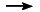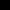HomeFunctional XPathSXPathSXPath Tutorial

(require (lib sxpath.ss sxml) (lib sxpathlib.ss sxml)) Textual, native and functional location steps in SXPath
Let's define a simple SXML element: (define e1 '(elt text)) and a simple SXML document which contains it: (define d1 `(*TOP* (element text1 ,e1 (nested (@ (attr value)) (element it_is_in_nested) text2 (deep deep1) (deep deep2) text5))))

This simple SXPath query

(define q1 (sxpath '(element nested))) or its textual XPath analog (define q1t (sxpath element/nested)) or the combination of low-level sxpath functions (define q1p (node-join (select-kids (ntype?? 'element)) (select-kids (ntype?? 'nested)))) may be used to select nested element from d1 document:
(q1 d1)
(q1t d1)
(q1p d1)

Native and textual location paths may be used together in one SXPath expression:

(define q2 (sxpath '(element nested/deep *text*)))

In the examples above SXPath queries were applied to SXML documents. If a query is applied to SXML node, some additional information is required for ascending axis processing

The query

(define q3 (sxpath ../text()) ) applied to the node e1 without any additional information
(q3 e1)
will yield an empty list because it considers the given node as the root node of the queried document.

This query will yield (text1) if the root of the queried document is specified:
(q3 e1 d1)

Context of XPath includes a set of variable bindings. Such the bindings may be provided as a list of (name . value) pairs and used in SXPath expression:
(q4 e1 d1 '((x . 2)))

(define q4 (sxpath ../nested/deep[\$x]) )

Please note that XPath 1.0 doesn't discuss processing of unbinded variables, but the latest Working Draft 15 November 2002 of XPath 2.0 says that an expression containing an unbound variable raises an error. So, for compatibility reasons current SXPath signals an error for (q4 e1 d1 '())

Any nodeset root var-bindings -> nodeset function may be used as a location step function in SXPath. For example, query q3 may be programmed as:

(define sf1 (lambda(node root vars) ((select-kids (ntype?? '*text*)) node))) (define q3p (sxpath `(.. ,sf1)))

This functions may make a use of XPath variables:

(define sf2 (lambda(node root vars) (let ((x (cdr (assq 'x vars)))) ((node-pos x) node)))) (define q4p (sxpath `(../nested/deep ,sf2))) or utilize root parameter: (define sf3 (lambda(node root vars) (let ((x (cdr (assq 'x vars)))) (((sxml:parent (ntype?? '*any*)) root) node)))) (define q4p2 (sxpath `(,sf3 nested deep ,sf2)))

SXParg query can combine location step functions, textual and native XPath notation:

(define q5 (sxpath `(,sf3 nested/deep ,sf2 *text*))) (q5 e1 d1 '((x . 2)))HomeFunctional XPathSXPath